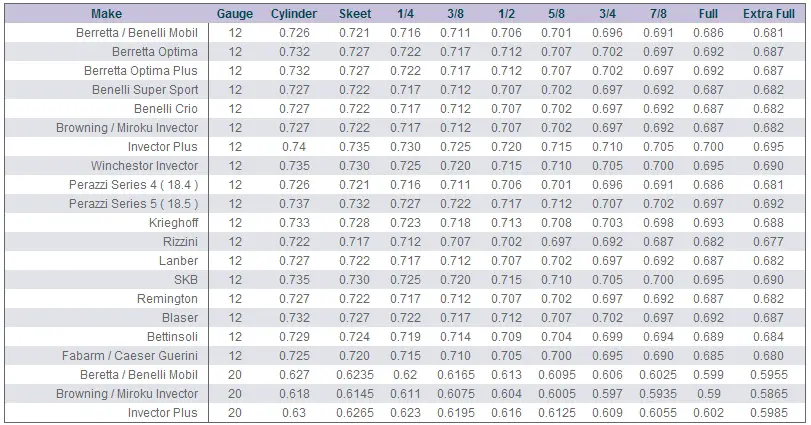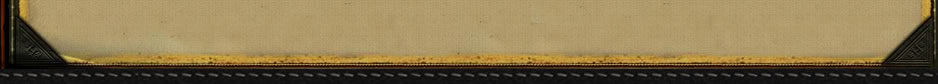Choke Constriction Reference Specifications for Krieghoff Factory Choke Tubes 12 Gauge - Available in standard Factory Steel and optional Titanium 00 = C = Cylinder = .000" constriction 0 = S = Skeet = .005" constriction 1 = IC = Improved Cylinder = .010" constriction 1+ = LM = Light Modified = .015" constriction 2 = M = Modified = .020" constriction 2+ = LIM = Light Improved Modified = .025" constriction 3 = IM = Improved Modified = .030" constriction 4 = F = Full = .035" constriction 20 Gauge - Available in standard Factory Steel and optional Titanium 00 = C = Cylinder = .000" constriction 0 = S = Skeet = .005" constriction 1 = IC = Improved Cylinder = .010" constriction 1+ = LM = Light Modified = .015" constriction 2 = M = Modified = .020" constriction 2+ = LIM = Light Improved Modified = .025" constriction 3 = IM = Improved Modified = .030" constriction 4 = F = Full = .035" constriction 28 Gauge - Available in standard Factory Steel only 00 = C = Cylinder = .000" constriction 0 = S = Skeet = .005" constriction 1 = IC = Improved Cylinder = .010" constriction 1+ = LM = Light Modified = .015" constriction 2 = M = Modified = .020" constriction 2+ = LIM = Light Improved Modified = .025" constriction 3 = IM = Improved Modified = .030" constriction 4 = F = Full = .035" constriction TRULOCK CHOKE CONSTRICTIONSNigel Teague, Teague Precision Chokes, LTDCopyright 2012 by Randy Wakeman. All Rights Reserved.Custom SearchCustom Search